##### Geometry For Dummies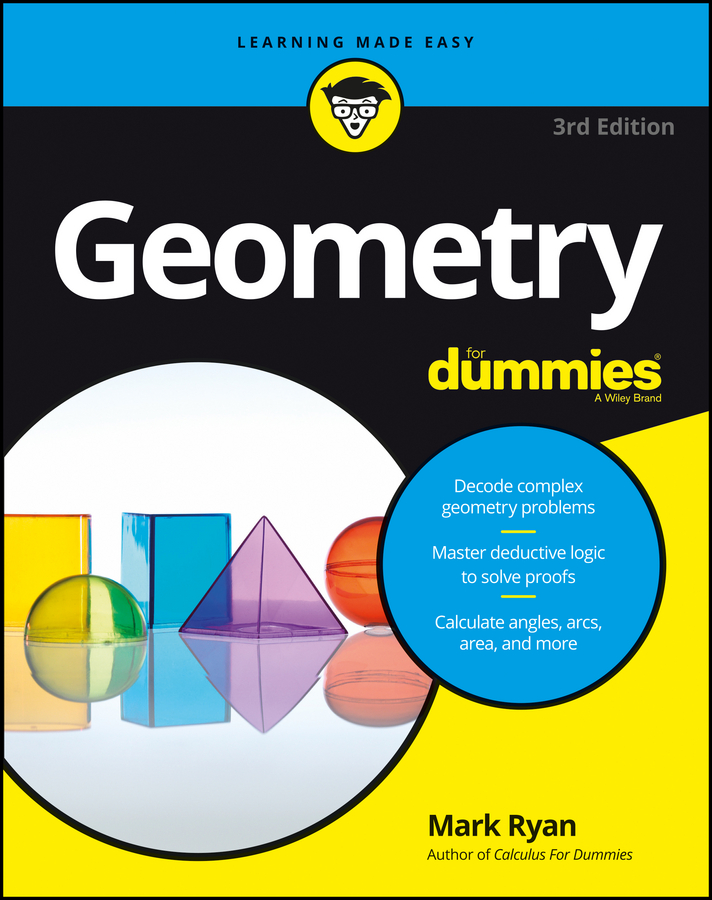To find the measure or size of a segment, you simply measure its length. What else could you measure? After all, length is the only feature a segment has. You’ve got your short, your medium, and your long segments. (No, these are not technical math terms.) Get ready for another shock: If you’re told that one segment has a length of 10 and another has a length of 20, then the 20-unit segment is twice as long as the 10-unit segment. Fascinating stuff, right?

Whenever you look at a diagram in a geometry book, paying attention to the sizes of the segments and angles that make up a shape can help you understand some of the shape’s important properties.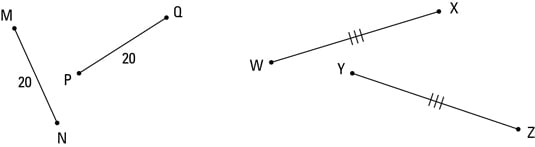Congruent segments are segments with the same length.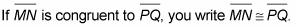You know that two segments are congruent when you know that they both have the same numerical length or when you don’t know their lengths but you figure out (or are simply told) that they’re congruent. In a figure, giving different segment the same number of tick marks indicates that they’re congruent.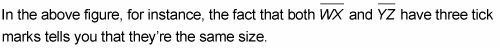Congruent segments are essential ingredients in proofs. For instance, when you figure out that a side (a segment) of one triangle is congruent to a side of another triangle, you can use that fact to help you prove that the triangles are congruent to each other.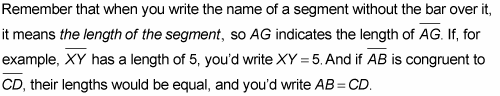Note that in the two preceding equations, an equal sign is used, not a congruence symbol.